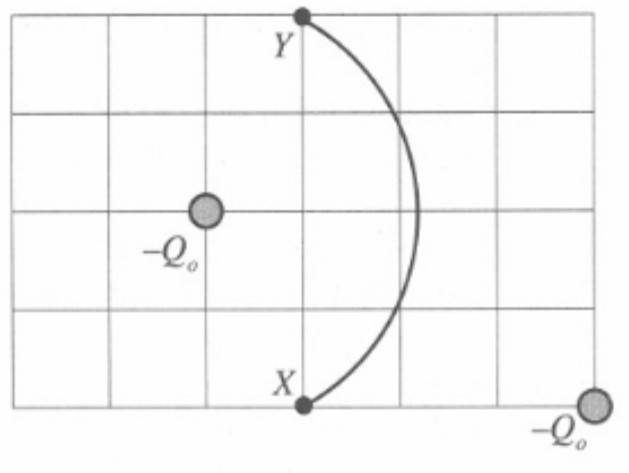# Problem: Two charged rods, each with net charge -Qo, are held in place as shown in the top view diagram below. A small test charge -qo travels from point X to point Y along the circular are shown. Draw an arrow on the diagram at each point (X and Y) to show the direction of the electric force on the test charge at that point. Explain why you drew the arrows as you did.

###### FREE Expert Solution

The electric field lines point outward from a positive charge and inward into a negative charge. Thus, the electric field points toward each rod.

The force experienced by negative charges points in a direction opposite to the direction of the electric field.

85% (105 ratings)###### Problem Details

Two charged rods, each with net charge -Qo, are held in place as shown in the top view diagram below.A small test charge -qo travels from point X to point Y along the circular are shown.

Draw an arrow on the diagram at each point (X and Y) to show the direction of the electric force on the test charge at that point. Explain why you drew the arrows as you did.